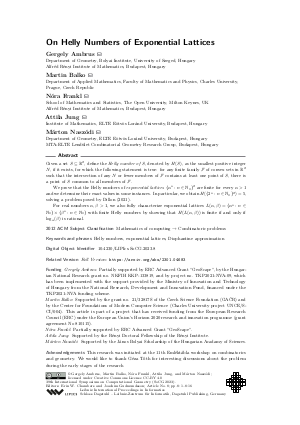Document# On Helly Numbers of Exponential Lattices

### Authors Gergely Ambrus, Martin Balko, Nóra Frankl, Attila Jung, Márton Naszódi## File

LIPIcs.SoCG.2023.8.pdf
• Filesize: 0.85 MB
• 16 pages

## Acknowledgements

This research was initiated at the 11th Emléktábla workshop on combinatorics and geometry. We would like to thank Géza Tóth for interesting discussions about the problem during the early stages of the research

## Cite As

Gergely Ambrus, Martin Balko, Nóra Frankl, Attila Jung, and Márton Naszódi. On Helly Numbers of Exponential Lattices. In 39th International Symposium on Computational Geometry (SoCG 2023). Leibniz International Proceedings in Informatics (LIPIcs), Volume 258, pp. 8:1-8:16, Schloss Dagstuhl - Leibniz-Zentrum für Informatik (2023)
https://doi.org/10.4230/LIPIcs.SoCG.2023.8

## Abstract

Given a set S ⊆ ℝ², define the Helly number of S, denoted by H(S), as the smallest positive integer N, if it exists, for which the following statement is true: for any finite family ℱ of convex sets in ℝ² such that the intersection of any N or fewer members of ℱ contains at least one point of S, there is a point of S common to all members of ℱ. We prove that the Helly numbers of exponential lattices {αⁿ : n ∈ ℕ₀}² are finite for every α > 1 and we determine their exact values in some instances. In particular, we obtain H({2ⁿ : n ∈ ℕ₀}²) = 5, solving a problem posed by Dillon (2021). For real numbers α, β > 1, we also fully characterize exponential lattices L(α,β) = {αⁿ : n ∈ ℕ₀} × {βⁿ : n ∈ ℕ₀} with finite Helly numbers by showing that H(L(α,β)) is finite if and only if log_α(β) is rational.

## Subject Classification

##### ACM Subject Classification
• Mathematics of computing → Combinatoric problems
##### Keywords
• Helly numbers
• exponential lattices
• Diophantine approximation

## Metrics

• Access Statistics
• Total Accesses (updated on a weekly basis)
0

## References

1. Nina Amenta, Jesús A. De Loera, and Pablo Soberón. Helly’s theorem: new variations and applications. In Algebraic and geometric methods in discrete mathematics, volume 685 of Contemp. Math., pages 55-95. Amer. Math. Soc., Providence, RI, 2017. URL: https://doi.org/10.1090/conm/685.
2. Gennadiy Averkov, Bernardo González Merino, Ingo Paschke, Matthias Schymura, and Stefan Weltge. Tight bounds on discrete quantitative Helly numbers. Adv. in Appl. Math., 89:76-101, 2017. URL: https://doi.org/10.1016/j.aam.2017.04.003.
3. David E. Bell. A theorem concerning the integer lattice. Studies in Appl. Math., 56(2):187-188, 1976/77. URL: https://doi.org/10.1002/sapm1977562187.
4. Michele Conforti and Marco Di Summa. Maximal S-free convex sets and the Helly number. SIAM J. Discrete Math., 30(4):2206-2216, 2016. URL: https://doi.org/10.1137/16M1063484.
5. Jesús A. De Loera, Reuben N. La Haye, Déborah Oliveros, and Edgardo Roldán-Pensado. Helly numbers of algebraic subsets of ℝ^d and an extension of Doignon’s theorem. Adv. Geom., 17(4):473-482, 2017. URL: https://doi.org/10.1515/advgeom-2017-0028.
6. Jesús A. De Loera, Reuben N. La Haye, David Rolnick, and Pablo Soberón. Quantitative Tverberg theorems over lattices and other discrete sets. Discrete Comput. Geom., 58(2):435-448, 2017. URL: https://doi.org/10.1007/s00454-016-9858-3.
7. Travis Dillon. Discrete quantitative Helly-type theorems with boxes. Adv. in Appl. Math., 129:Paper No. 102217, 17, 2021. URL: https://doi.org/10.1016/j.aam.2021.102217.
8. Jean-Paul Doignon. Convexity in cristallographical lattices. J. Geom., 3:71-85, 1973. URL: https://doi.org/10.1007/BF01949705.
9. Alexey Garber. On Helly number for crystals and cut-and-project sets. Arxiv preprint https://arxiv.org/abs/1605.07881, 2017.
10. Jaroslav Hančl and Ondřej Turek. One-sided Diophantine approximations. Journal of Physics A: Mathematical and Theoretical, 52(4):045205, January 2019. URL: https://doi.org/10.1088/1751-8121/aaf5d3.
11. Eduard Helly. Über Mengen konvexer Körper mit gemeinschaftlichen Punkten. Jahresber. Deutsch. Math.-Verein., 32:175-176, 1923.12. Alan J. Hoffman. Binding constraints and Helly numbers. In Second International Conference on Combinatorial Mathematics (New York, 1978), volume 319 of Ann. New York Acad. Sci., pages 284-288. New York Acad. Sci., New York, 1979.13. Andreas Holmsen and Rephael Wenger. Helly-type theorems and geometric transversals. In Handbook of Discrete and Computational Geometry (3rd ed.). CRC Press, 2017.14. Aleksandr Ya. Khinchin. Continued fractions. Dover Publications, Inc., Mineola, NY, Russian edition, 1997. With a preface by B. V. Gnedenko, reprint of the 1964 translation.15. Herbert E. Scarf. An observation on the structure of production sets with indivisibilities. Proc. Nat. Acad. Sci. U.S.A., 74(9):3637-3641, 1977. URL: https://doi.org/10.1073/pnas.74.9.3637.
16. Kevin Barrett Summers. The Helly Number of the Prime-coordinate Point Set. Bachelor’s thesis, University of California, 2015.X

Feedback for Dagstuhl Publishing